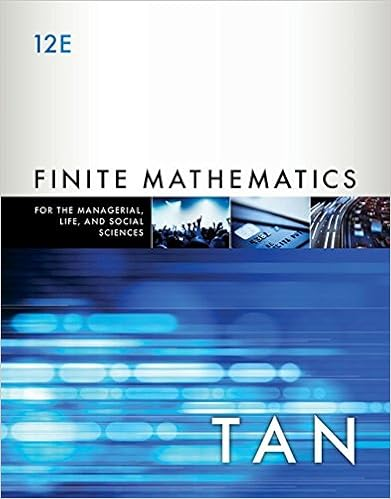# The depreciation is greatest in the first year and

• 122

This preview shows page 32 - 34 out of 122 pages.

##### We have textbook solutions for you!
The document you are viewing contains questions related to this textbook.The document you are viewing contains questions related to this textbook.
Chapter 5 / Exercise 40
Finite Mathematics for the Managerial, Life, and Social Sciences: An Applied Approach
TanExpert Verified
The depreciation is greatest in the first year and declines each year after that. Finally, the depreciation in the last year is limited to the amount necessary to reduce book value to residual value, Br. 2500 = Br.12,500 Br.10,000 (i.e. Previous book value minus residual value).Because, total depreciation over the life of the asset is limited to total depreciable cost/base. Fractional Period Depreciation Under this method, fractional years involve a very simple adaptation to the approach presented above. The first partial year will be a fraction of the annual amount, and all subsequent years will be the normal calculation (twice the straight-line rate times the beginning year book value). Assuming from previous example that the plant asset is purchased and put in use on April 1, year 1. The depreciation expense of each period would be: Year Depreciation expense * Accumulated depreciation Book value Year1 37,500 ( 100,000 * 50% * 9/12 ) 37,500 62,500 Year2 31,250 ( 100,000 - \$37,500 * 50%) 68,750 31,250 Year3 15,625( 100,000 - \$68,750 * 50%) 84,375 15,625
##### We have textbook solutions for you!
The document you are viewing contains questions related to this textbook.The document you are viewing contains questions related to this textbook.
Chapter 5 / Exercise 40
Finite Mathematics for the Managerial, Life, and Social Sciences: An Applied Approach
TanExpert Verified
33 | P a g e Compiled by: Mekonnen K. (M.Sc in Accounting and Finance) 2017 Year4 5,625 ( 100,000 - \$84,375 * 50%) or 15625 10,000 (salvage value) 90,000 10,000 4. The Sum of Years Digits Method Like the declining balance method, the sum of the year‟s digits method also provides a higher amount of periodic depreciation expense in the earlier use of the asset and declining depreciation expense thereafter. This method takes the anticipated life of the asset as the basis and weights its years most heavily. Under this technique, depreciation expense for a given year is determined by multiplying the depreciable base by a fraction; the numerator is a digit relating to the year of use and the denominator is the sum of the year‟s digits. For example, if there are 5 years in the asset‟s life, the sum of the digits is 15 (5 + 4 + 3 + 2 +1). In the first year 5/15 th of the depreciable cost of the asset is charged for depreciation. In the 2 nd year 4/15 th , and so on until the final year has 1/15 th charged for depreciation. To save time in adding up the digits for each asset each year, use the following shortcut to compute the denominator: Denominator = n (n+1) 2 Where “n” is the useful life of the asset. = 5 (5+1)/2 = 30/2 = 15 On the other hand, the numerator of the fraction is the digits representing useful life of the asset in reverse order or years remaining in the assets life as of the beginning of the year. For example, if you are depreciating an asset with a 10-year estimated life, the numerator to use at the end of Year 1 is 10 because that is the number of years remaining in the asset‟s life as of the beginning of the first year. The numerator to use at the end of Year 2 is 9, because that is the number of years remaining in the asset‟s life as of the beginning of Year 2. Because the numerator changes each year, the SYD depreciation rate also changes each year.
•••